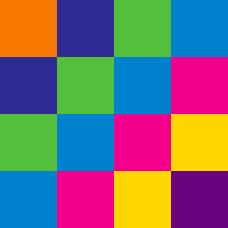Basic Mathematics

# Pattern Recognition: Level 3 Challenges

$\large 1, 2, 2, 3, 3, 3, 4, 4, 4, 4, 5, 5, 5, 5, 5, \ldots$

What is the 1000th term in the sequence above?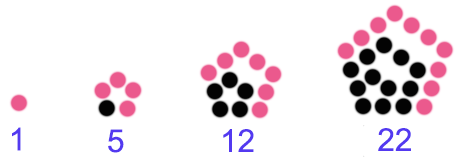The above shows the first 4 pentagonal numbers, namely 1, 5, 12, 22.

How many points need to be added to the $36^\text{th}$ pentagonal number to get the $37^\text{th}$ pentagonal number?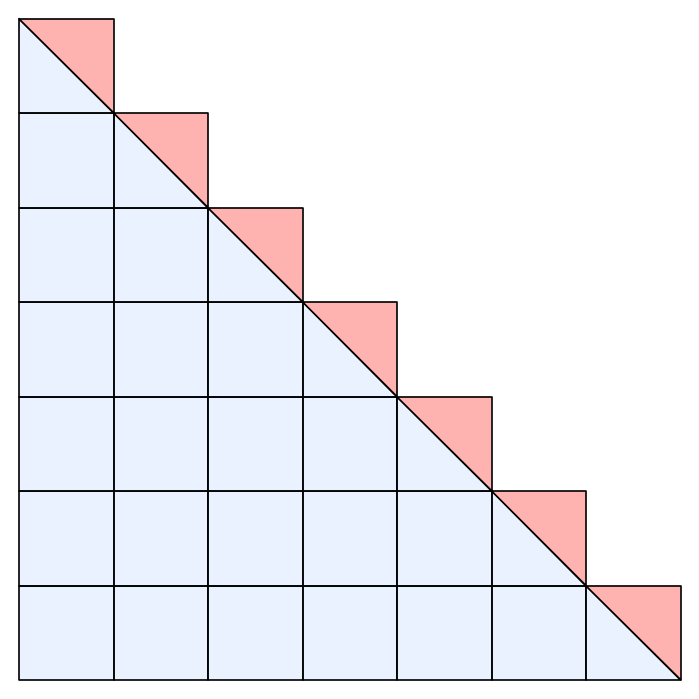The picture above illustrates which of the following: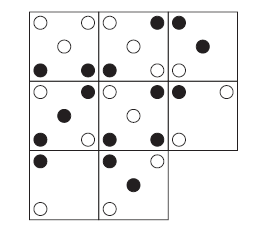Which is the missing square ? Assume that "blank" added to anything else results in a "blank".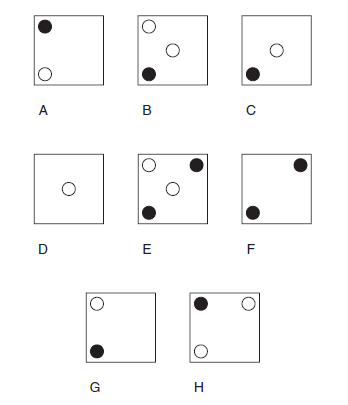$\begin{array}{c}& & & & 1& & \\ & & 2 & 3 & 4 & & \\ & 5 & 6 & 7 & 8 & 9 & \\ 10 & 11 & 12 & 13 & 14 & 15 & 16 \\ &&& \vdots &&& \end{array}$

Given that the pattern continues, find the second term in the $13^\text{th}$ row.

×